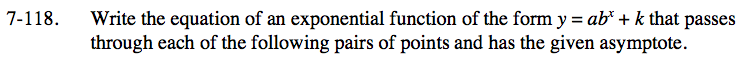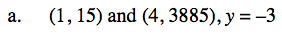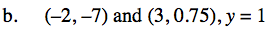Home > INT3 > Chapter 7 > Lesson 7.2.5 > Problem7-118

7-118.

Write the equation of an exponential function of the form y = abx + k that passes through each of the following pairs of points and has the given asymptote. Homework Help ✎

1. (1, 15) and (4, 3885), y = –3

2. (–2, –7) and (3, 0.75), y = 1Write two equations by substituting the coordinates for x and y.

15 = ab1 − 3
3885 = ab4 − 3

Use substitution to find the value of a and b. Start by adding 3 to both sides of the equations.

$a = \frac{18}{b}$

$3888 = \frac{18}{b}b^4$

Solve for b. Then solve for a.

y = 3(6)x − 3Use the same method as part (a).